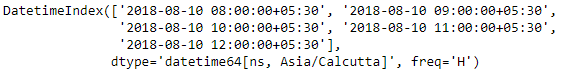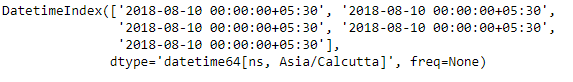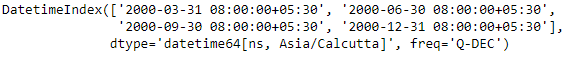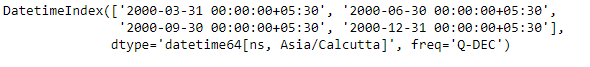# Python | Pandas DatetimeIndex.normalize()

Python is a great language for doing data analysis, primarily because of the fantastic ecosystem of data-centric python packages. Pandas is one of those packages and makes importing and analyzing data much easier.

Pandas` DatetimeIndex.normalize()` function convert times to midnight. The time component of the date-timeise converted to midnight i.e. 00:00:00. This is useful in cases, when the time does not matter. Length is unaltered. The timezones are unaffected.

Syntax: DatetimeIndex.normalize()

Return: DatetimeIndex or Series

Example #1: Use `DatetimeIndex.normalize()` function to normalize time.

 `# importing pandas as pd ` `import` `pandas as pd ` ` `  `# Create the DatetimeIndex ` `# Here 'H' represents hourly frequency  ` `idx ``=` `pd.DatetimeIndex(start ``=``'2018-08-10 08:00'``, freq ``=``'H'``,  ` `                           ``periods ``=` `5``, tz ``=``'Asia/Calcutta'``) ` ` `  `# Print the DatetimeIndex ` `print``(didx) `

Output :Now we want all the time values present in the DatetimeIndex object to be normalized i.e., it gets converted to midnight time.

 `# normalize the time. ` `idx.normalize() `

Output :As we can see in the output, the function has converted all the time values in the object to midnight time.

Example #2: Use `DatetimeIndex.normalize()` function to normalize time.

 `# importing pandas as pd ` `import` `pandas as pd ` ` `  `# Create the DatetimeIndex ` `# Here 'Q' represents quarter end frequency  ` `idx ``=` `pd.DatetimeIndex(start ``=``'2000-01-15 08:00'``, freq ``=``'Q'``,  ` `                           ``periods ``=` `4``, tz ``=``'Asia/Calcutta'``) ` ` `  `# Print the DatetimeIndex ` `print``(didx) `

Output :Now we want all the time values present in the DatetimeIndex object to be normalized i.e., it gets converted to midnight time.

 `# normalize the time. ` `idx.normalize() `

Output :As we can see in the output, the function has converted all the time values in the object to midnight time.

Attention geek! Strengthen your foundations with the Python Programming Foundation Course and learn the basics.

To begin with, your interview preparations Enhance your Data Structures concepts with the Python DS Course.

My Personal Notes arrow_drop_upCheck out this Author's contributed articles.

If you like GeeksforGeeks and would like to contribute, you can also write an article using contribute.geeksforgeeks.org or mail your article to contribute@geeksforgeeks.org. See your article appearing on the GeeksforGeeks main page and help other Geeks.

Please Improve this article if you find anything incorrect by clicking on the "Improve Article" button below.

Article Tags :

Be the First to upvote.

Please write to us at contribute@geeksforgeeks.org to report any issue with the above content.